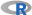﻿ 5. Detailed description of the Actions > 5.11. R Predictive > 5.11.8. Exponential Smoothing (R action)

# 5.11.8. Exponential Smoothing (action)

Icon:Function: expSmoothing

Property window:Short description:

Exponential Smoothing.

Long Description:

This action is useful for time serie forecasting. It allows three algoritms: two (STFL and ETS) are from the “Exponential Smoothing” family (so is the “Holt-Winters” algorithm), the third is a classical ARIMA.

Parameters:

Unit of Time : specify the interval between the observations

Starting Year: specify the year of the first observation

Starting Unit of Time: specify the period of the first observation (e.g.: for Month: February = 2, for Quarter:  Jul-Sep = 3, ...)

Prediction length to forecast : how many periods to forecast in the future

Forecasting Algorithm : what type of algorithm to use for computing the forecast, with hyper parameters automaticaly optimized using AIC criteria :

ETS : Exponential smoothing state space model

STFL: Method applying a non-seasonal forecasting method to the seasonally adjusted data and re-seasonalizing using the last year of the seasonal component

ARIMA : Autoregressive integrated moving average model

Optional:
Aggregate Time Serie to (Optional): If missing observations, or just for business need, specify the period on which to aggregate the original time serie. Days can therefore be aggregated to weeks, month, quarters or year; weeks to month, quarter or year, ...

Aggregation function (Optional): specify how to aggregate the values by period. Only sum and mean are available so far.

Plot Results: Click to plot the results. To adjust the title to the name of the series, simply change the partition type to “partition by Column”, the name of the plot will then be the name of the partition.

Data Source: the data shoulb be organized in ROWS, where each is a time series, and column represent equal time interval (which means, there are potential missing value issues).The following example first onverts the data to NUMBER (float), keeps only the relevant columns, filters the time series we wish to compute and runs the models.